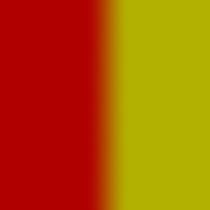### RSLColor Ramps

#### Introduction

This tutorial provides a couple of examples of shaders that apply a simple color ramp to a surface.

##### Simple Color Ramp

Using the `mix()` function a color can be assigned that changes smoothly in either the 's' or the 't' direction.Figure 1

Listing 1

 ```surface ramp1(float Kfb = 1; color left = color(1,0,0), right = color(1,1,0)) { color surfcolor = mix(left, right, s); Oi = Os; Ci = Oi * Cs * surfcolor * Kfb; }```

##### Color Step

Using the smoothstep() function the color of a surface can be made to change more or less abruptly at a specific location.Figure 2

Listing 2

 ```surface ramp2(float Kfb = 1, blur = 0.1, sdist = 0.5; color left = color(1,0,0), right = color(1,1,0)) { color surfcolor = mix(left, right, smoothstep(sdist-blur,sdist+blur,s)); Oi = Os; Ci = Oi * Cs * surfcolor * Kfb; }```